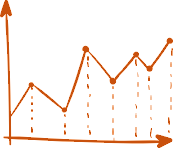# Analyzing Life Science data using XLStat

In this case, practical examples are processed with XLSTAT, which enable the participants to understand the background. The course expressly does not understand itself as a mathematically oriented course, which teaches the participants formula knowledge. Rather, it gives an overview of the different possibilities, application contexts and risks.

#### Analyzing Life Science data using XLStat

This course expressly is not theoretical nor mathematical, so we will not focus on formulae but on idea, areas of application and risks of the procedures.

Contents:
• Basic Statistics - descriptive statistics using XLSTAT
• statistical graphics
- what is a good graph? barcharts, error bars, Dose-Response Curves using XLSAT
• setup and test of statistical hypothesis in XLSTAT
- simple t-Test
- ANOVA (Analysis of variance) Repeated Measures
- Non-parametric tests e.g. Mann-Whiteny-U, Kruskal- Wallis-Wilcoxon- and Friedman-Test
- linear and nonlineare Regression including Dose- Response Curves
- data transformation and analyzing residuals (checking assumptions: homogeneity of variances, normality and Lack-of-Fit
- proper setup of experiments, DoE
• Survival analysis in XLSTAT - Kaplan-Meier Curves, Cox and Parametric Survival models

1.470,00 €
por persona

735,00 €
por persona

Inicio:

Fin:

Alemán

#### Localización

Witzenhausen Germany